# 十二、深度学习简介

• 计算机视觉任务的深度学习概述
• OpenCV 中的深度学习
• TensorFlow 库
• Keras 库

# 技术要求

• Python 和 OpenCV
• 特定于 Python 的 IDE
• NumPy 和 Matplotlib 包
• Git 客户端
• TensorFlow 库（请参阅以下有关如何安装 TensorFlow 的部分）
• Keras 库（请参阅以下有关如何安装 Keras 的部分）

$pip install tensorflow  要检查安装是否已正确执行，只需打开 Python shell 并尝试导入 TensorFlow 库，如下所示： python import tensorflow  # 安装 Keras 为了安装 Keras，请使用以下命令： $ pip install keras


python
import keras


# 深度学习特征

• 与可以在低端机器上运行的机器学习技术相反，深度学习算法需要具有高端基础架构才能正确训练。 实际上，深度学习算法固有地执行了大量计算，而这些计算可以使用 GPU 进行优化。
• 当对特征自省和工程都缺乏领域的了解时，深度学习技术会胜过其他技术，因为您不必担心特征工程。 特征工程可以定义为将领域知识应用于特征检测器和提取器创建的过程，目的是降低数据的复杂性，从而使传统的机器学习方法能够正确学习。 因此，这些机器学习算法的表现取决于识别和提取特征的准确率。 另一方面，深度学习技术试图从数据中提取高级特征，这使得深度学习比传统的机器学习方法先进得多。 在深度学习中，查找相关特征的任务是算法的一部分，并且通过减少每个问题的特征自省和工程任务来实现自动化。
• 机器学习和深度学习都能够处理海量数据集。 但是，在处理小型数据集时，机器学习方法更有意义。 从这个意义上说，这两种方法之间的主要区别在于其表现随着数据规模的增加而增加。 例如，当使用小型数据集时，深度学习算法很难在数据中找到模式，并且表现不佳，因为它们需要大量数据来调整其内部参数。 经验法则是，如果数据量很大，则深度学习要胜过其他技术，而当数据集较小时，传统的机器学习算法是可取的。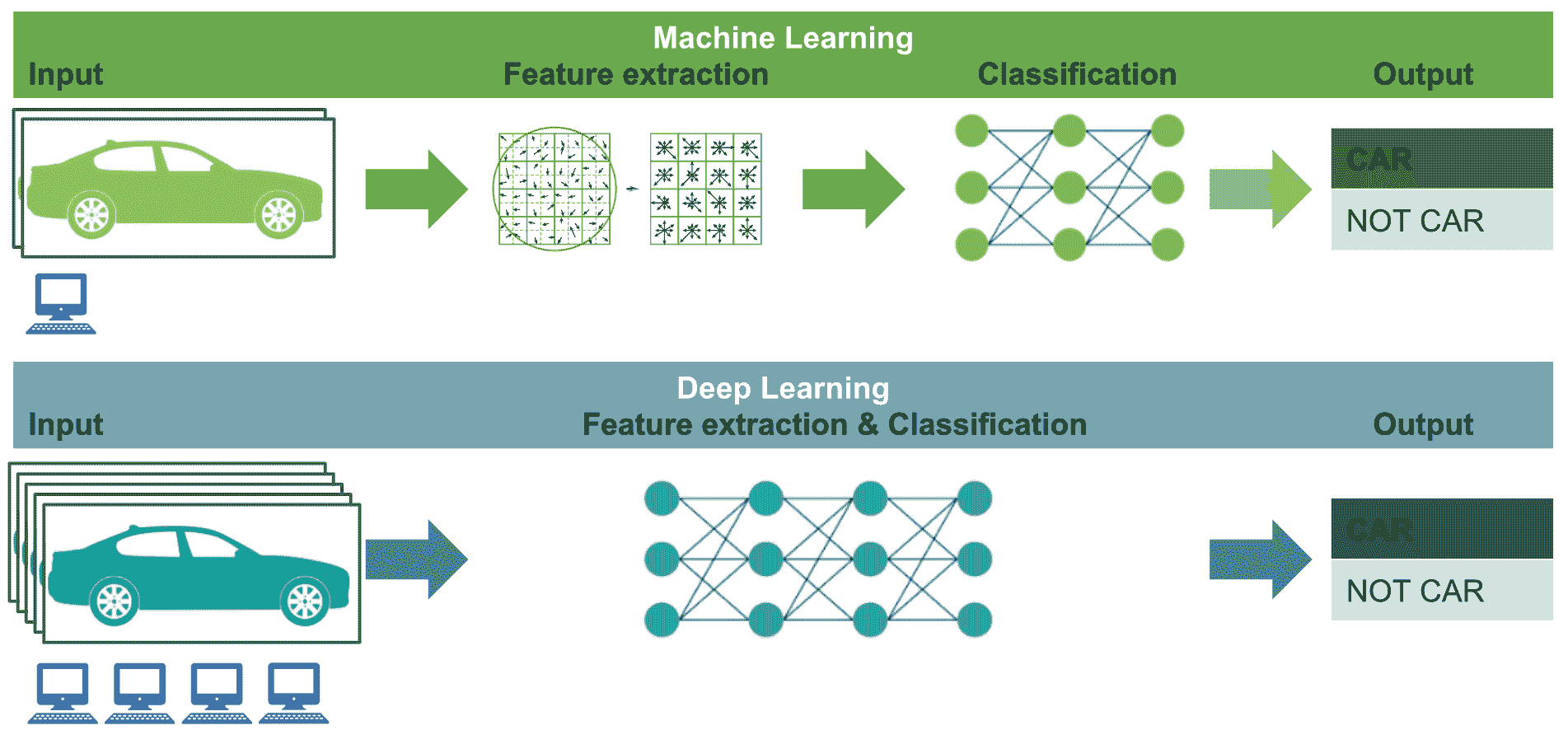• 计算资源（深度学习–高端机器与机器学习–低端机器）
• 特征工程（深度学习–同一步骤中的特征提取和分类与机器学习–单独步骤中的特征提取和分类）
• 数据集大小（深度学习–大/非常大的数据集与机器学习–中/大数据集）

# 用于图像分类的深度学习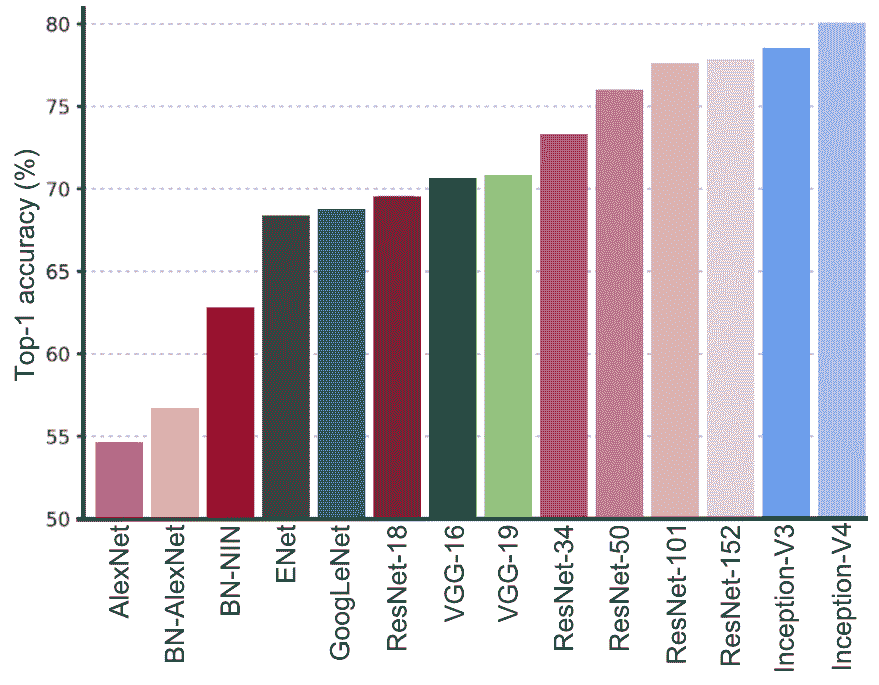• AlexNet（2012）
• 描述：AlexNet 是 LSVRC-2012 的获胜者，它是一种简单但功能强大的网络架构，其中卷积层和池层一个接一个，而顶层则是全连接层。 在将深度学习方法应用于计算机视觉任务时，通常将该架构用作起点。
• 参考Alex Krizhevsky, Ilya Sutskever, and Geoffrey E Hinton. ImageNet classification with deep convolutional neural networks. In Advances in neural information processing systems, pp. 1097–1105, 2012.
• VGG-16 和 -19（2014）
• 描述：VGGNet 由牛津大学的视觉几何组VGG）提出。 通过在整个网络中仅使用3 x 3过滤器，而不使用大型过滤器（例如7 x 711 x 11）。 这项工作的主要贡献在于，它表明网络深度是在卷积神经网络中实现更好的识别或分类精度的关键组成部分。 VGGNet 被认为是对特定任务进行基准测试的良好架构。 但是，它的主要缺点是训练速度非常慢，并且网络架构的权重很大（VGG-16 为 533 MB，VGG-19 为 574 MB）。 VGGNet-19 使用 1.38 亿个参数。
• 参考Simonyan, K., and Zisserman, A. (2014). Very deep convolutional networks for large-scale image recognition. arXiv preprint arXiv:1409.1556.
• 说明GoogLeNet（也称为 Inception V1）是 LSVRC-2014 的获胜者，其前 5 名错误率达到 6.67%，这非常接近人类水平的表现。 该架构比 VGGNet 更深入。 但是，由于 9 个并行模块（初始模块）的架构是基于几个非常小的卷积的，因此它仅使用 AlexNet 参数数量的十分之一（从 6000 万到仅 400 万个参数），目的是减少参数数量。
• 参考Szegedy, C., Liu, W., Jia, Y., Sermanet, P., Reed, S., Anguelov, D., Dumitru, .E, Vincent, .V, and Rabinovich, A. (2015). Going deeper with convolutions.
• ResNet-18，-34，-50，-101 和 -152（2015）
• 说明：Microsoft 的残差网络ResNets）是 LSVRC-2015 的获胜者，并且是迄今为止最深的网络，共有 153 个卷积层达到了最高 5 个分类误差为 4.9%（这比人工精度略好）。 此架构包括跳跃连接，也称为门控单元门控循环单元，可实现增量学习更改。 ResNet-34 使用 2180 万个参数，ResNet-50 使用 2560 万个参数，ResNet-101 使用 4450 万个参数，最后，ResNet-152 使用 6020 万个参数。
• 参考He, K., Zhang, X., Ren, S., and Sun, J. (2016). Deep residual learning for image recognition. In Proceedings of the IEEE conference on computer vision and pattern recognition (pp. 770-778).
• Inception V3（2015）

• 描述：如前所示，初始架构被引入为 GoogLeNet（也称为 Inception V1）。 后来，对该架构进行了修改，以引入批量规范化（Inception-V2）。 Inception V3 架构包括其他分解思想，其目的是在不降低网络效率的情况下减少连接/参数的数量。
• 参考Szegedy, C., Vanhoucke, V., Ioffe, S., Shlens, J., and Wojna, Z. (2016). Rethinking the inception architecture for computer vision. In Proceedings of the IEEE conference on computer vision and pattern recognition (pp. 2818-2826).
• Inception V4（2016）

• 说明：从 GoogLeNet 演变而来的 Inception V4。 另外，与 Inception-V3 相比，此架构具有更统一的简化架构和更多的 Inception 模块。 Inception-V4 在 LSVRC 上能够达到 80.2% 的 top-1 精度和 95.2% 的 top-5 精度。
• 参考Szegedy, C., Ioffe, S., Vanhoucke, V., and Alemi, A. A. (2017, February). Inception-V4, inception-resnet and the impact of residual connections on learning. In AAAI (Vol. 4, p. 12).

# 用于对象检测的深度学习

• PASCAL VOC2007 挑战–仅考虑了一个 IoU 阈值。 对于 PASCAL VOC 挑战，如果 IoU> 0.5，则预测为肯定。 因此，mAP 是对所有 20 个对象类平均的。
• 在 2017 年 COCO 挑战赛中，对所有 80 个物体类别和所有 10 个 IoU 阈值（从 0.5 到 0.95，步长为 0.05）平均了 mAP。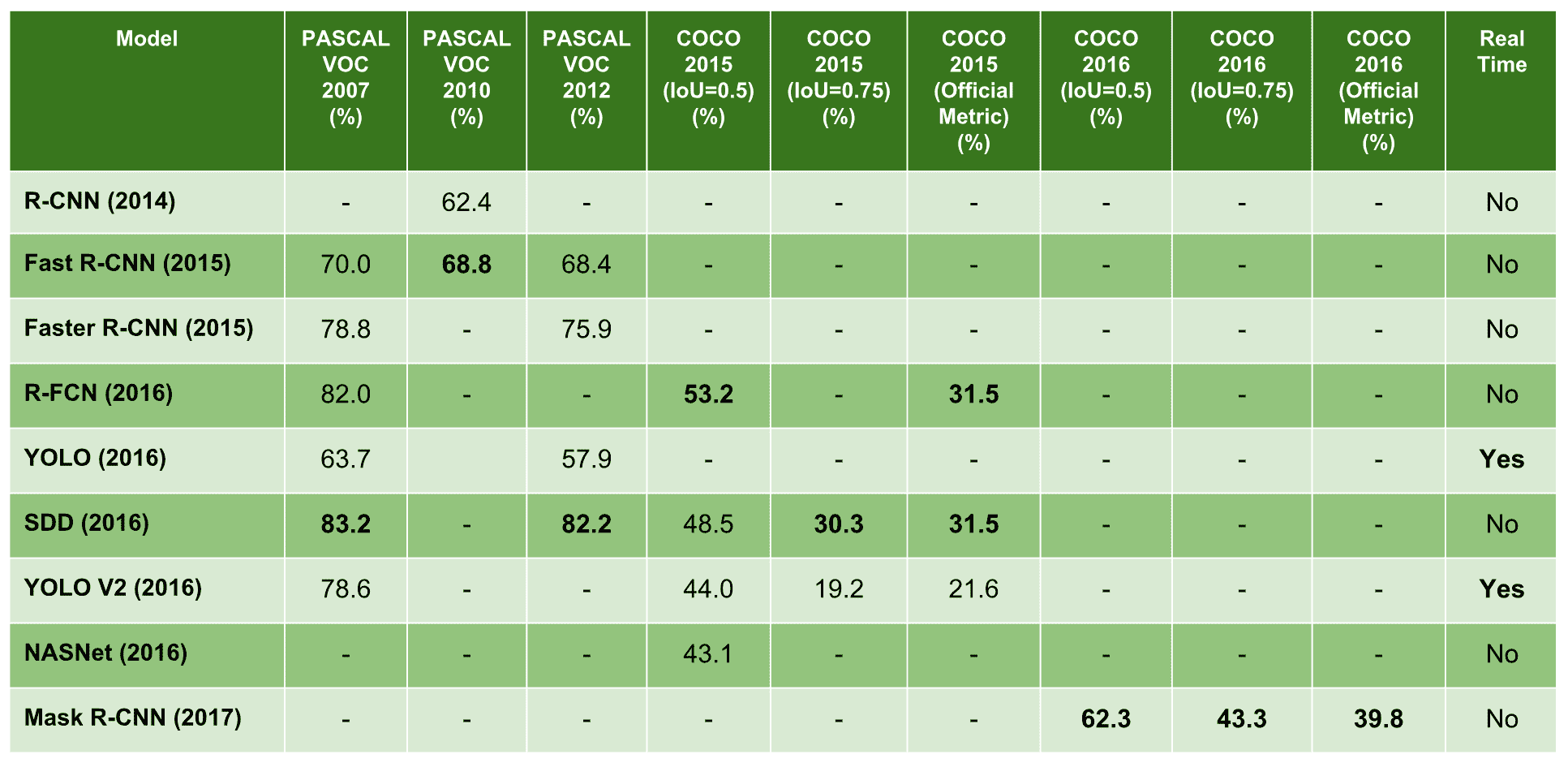• R-CNN（2014）
• 描述基于区域的卷积网络R-CNN）是使用卷积神经网络进行对象检测的首批方法之一，表明与基于类似 HOG 的简单特征的系统相比，卷积神经网络可以提高目标检测表现。 该算法可以分解为以下三个步骤：
1. 创建一组区域提议
2. 对每个区域提议执行经过修订版的 AlexNet 的前向传递，以提取特征向量
3. 潜在的对象通过几个 SVM 分类器进行检测，此外，线性回归器会更改边界框的坐标
• 参考Girshick, R., Donahue, J., Darrell, T., and Malik, J. (2014). Rich feature hierarchies for accurate object detection and semantic segmentation. In Proceedings of the IEEE conference on computer vision and pattern recognition (pp. 580-587).
• Fast R-CNN（2015）
• 描述基于快速区域的卷积网络Fast R-CNN）方法是对先前方法的一种改进，可以有效地对目标提议进行分类。 此外，Fast R-CNN 采用了多项创新技术来提高训练和测试速度，同时还提高了检测精度。
• 参考Girshick, R. (2015). Fast r-cnn. In Proceedings of the IEEE international conference on computer vision and pattern recognition (pp. 1440-1448).
• Faster R-CNN（2015）
• 说明：更快的 R-CNN 是对快速 R-CNN 的修改，引入了一个区域提议网络RPN），该网络与检测网络共享全图像卷积特征，从而实现几乎免费的区域提议。
• 参考Ren, S., He, K., Girshick, R., and Sun, J. (2015). Faster R-CNN – Towards real-time object detection with region proposal networks. In Advances in neural information processing systems (pp. 91-99).
• R-FCN（2016）
• 描述基于区域的全卷积网络R-FCN）是仅包含卷积层的框架，允许进行完整的反向传播以进行训练和推理，从而获得准确而有效的对象检测。
• 参考Dai, J., Li, Y., He, K., and Sun, J. (2016). R-FCN: Object Detection via Region-based Fully Convolutional Networks. In Advances in neural information processing systems (pp. 379-387).
• YOLO（2016）
• 描述只看一次YOLO）是一种深度学习架构，可在单个步骤中预测边界框和类概率。 与其他深度学习检测器相比，YOLO 会产生更多的定位错误，但是在背景中预测假正例的可能性较小。
• 参考Redmon, J., Divvala, S., Girshick, R., and Farhadi, A. (2016). You only look once: Unified, Real-Time Object Detection.
• SSD（2016）
• 描述单发多盒检测器SSD）是一个深层神经网络，旨在通过端到端卷积神经网络架构的方法，同时预测边界框和类概率。
• 参考Liu, W., Anguelov, D., Erhan, D., Szegedy, C., Reed, S., Fu, C. Y., and Berg, A. C. (2016, October). SSD: Single Shot Multibox Detector. In European conference on Computer Vision (pp. 21-37). Springer, Cham.
• YOLO V2（2016）
• 描述：作者在同一出版物中介绍了 YOLO9000 和 YOLO V2。 YOLO9000 是一种实时对象检测系统，可以检测 9,000 多个对象类别，而 YOLO V2 是 YOLO 的改进版本，致力于在提高准确率的同时仍是快速检测器。
• 参考Redmon, J., and Farhadi, A. (2017). YOLO9000: Better, Faster, Stronger. arXiv preprint.
• NASNet（2016）
• 描述：作者介绍了一种神经网络搜索，这是使用循环神经网络构成神经网络架构的想法。 神经架构搜索网络NASNet）包括学习模型的架构，以优化层数，同时还提高准确率。
• 参考Zoph, B., and Le, Q. V. (2016). Neural Architecture Search with Reinforcement Learning. arXiv preprint arXiv:1611.01578.
• 描述基于遮罩区域的卷积网络遮罩 R-CNN）是 Faster R-CNN 模型的另一个扩展，它为边界框检测添加了并行分支，目的是预测对象遮罩。 对象遮罩是按图像中的像素进行分割，从而可以对对象实例进行分割。
• 参考He, K., Gkioxari, G., Dollár, P., and Girshick, R. (2017, October). Mask r-cnn. In Computer Vision (ICCV), 2017 IEEE International Conference on Computer Vision (pp. 2980-2988). IEEE.

# 了解cv2.dnn.blobFromImage()

net = cv2.dnn.readNetFromCaffe("deploy.prototxt", "res10_300x300_ssd_iter_140000_fp16.caffemodel")


# Load image:

# Create 4-dimensional blob from image:
blob = cv2.dnn.blobFromImage(image, 1.0, (300, 300), [104., 117., 123.], False, False)


| 模型 | 规模 | 尺寸WxH | 均值减法 | 通道顺序 | | --- | --- | --- | --- | | OpenCV 人脸检测器 | 1.0 |300 x 300| 104177123 | BGR |

# Set the blob as input and obtain the detections:
net.setInput(blob)
detections = net.forward()


cv2.dnn.blobFromImage()的签名如下：

retval=cv2.dnn.blobFromImage(image[, scalefactor[, size[, mean[, swapRB[, crop[, ddepth]]]]]])


• image：这是要预处理的输入图像。
• scalefactor：这是image值的乘数。 此值可用于缩放我们的图像。 默认值为1.0，这表示不执行缩放。
• size：这是输出图像的空间大小。
• mean：这是从图像中减去平均值的标量。 如果执行均值减法，则在使用swapRB =True时，这些值应为（mean-Rmean-Gmean-B）。
• swapRB：通过将该标志设置为True，可以使用该标志交换图像中的RB通道。
• crop：这是一个标志，指示在调整大小后是否将裁切图像。
• ddepth：输出 BLOB 的深度。 您可以在CV_32FCV_8U之间选择。
• 如果为crop=False，则在不裁剪的情况下执行图像的调整大小。 否则，如果（crop=True），则首先应用调整大小，然后从中心裁剪图像。
• 默认值为scalefactor=1.0size = Size()mean = Scalar()swapRB = falsecrop = falseddepth = CV_32F

cv.dnn.blobFromImages()的签名如下：

retval=cv.dnn.blobFromImages(images[, scalefactor[, size[, mean[, swapRB[, crop[, ddepth]]]]]])


# Create a list of images:
images = [image, image2]

# Call cv2.dnn.blobFromImages():
blob_images = cv2.dnn.blobFromImages(images, 1.0, (300, 300), [104., 117., 123.], False, False)

# Set the blob as input and obtain the detections:
net.setInput(blob_images)
detections = net.forward()


blob_from_image.py脚本中，我们首先加载 BGR 图像，然后使用cv2.dnn.blobFromImage()函数创建一个二维 BLOB。 您可以检查创建的 BLOB 的形状是否为(1, 3, 300, 300)。 然后，我们调用get_image_from_blob()函数，该函数可用于执行逆预处理转换，以便再次获取输入图像。 这样，您将更好地了解此预处理。 get_image_from_blob函数的代码如下：

def get_image_from_blob(blob_img, scalefactor, dim, mean, swap_rb, mean_added):
"""Returns image from blob assuming that the blob is from only one image""
images_from_blob = cv2.dnn.imagesFromBlob(blob_img)
image_from_blob = np.reshape(images_from_blob, dim) / scalefactor
image_from_blob_mean = np.uint8(image_from_blob)
image_from_blob = image_from_blob_mean + np.uint8(mean)

if swap_rb:
image_from_blob = image_from_blob[:, :, ::-1]
return image_from_blob
else:
if swap_rb:
image_from_blob_mean = image_from_blob_mean[:, :, ::-1]
return image_from_blob_mean


# Load image:

# Call cv2.dnn.blobFromImage():
blob_image = cv2.dnn.blobFromImage(image, 1.0, (300, 300), [104., 117., 123.], False, False)

# The shape of the blob_image will be (1, 3, 300, 300):
print(blob_image.shape)

# Get different images from the blob:
img_from_blob = get_image_from_blob(blob_image, 1.0, (300, 300, 3), [104., 117., 123.], False, True)
img_from_blob_swap = get_image_from_blob(blob_image, 1.0, (300, 300, 3), [104., 117., 123.], True, True)
img_from_blob_mean = get_image_from_blob(blob_image, 1.0, (300, 300, 3), [104., 117., 123.], False, False)
img_from_blob_mean_swap = get_image_from_blob(blob_image, 1.0, (300, 300, 3), [104., 117., 123.], True, False)


• img_from_blob图像对应于调整为(300,300)的原始 BGR 图像。
• img_from_blob_swap图像对应于调整为(300,300)尺寸的原始 BGR 图像，并且蓝色和红色通道已交换。
• img_from_blob_mean图像对应于调整为(300,300)尺寸的原始 BGR 图像，其中未将具有平均值的标量添加到图像中。
• img_from_blob_mean_swap图像对应于调整为(300,300)的原始 BGR 图像，其中未将具有平均值的标量添加到该图像，并且已交换了蓝色和红色通道。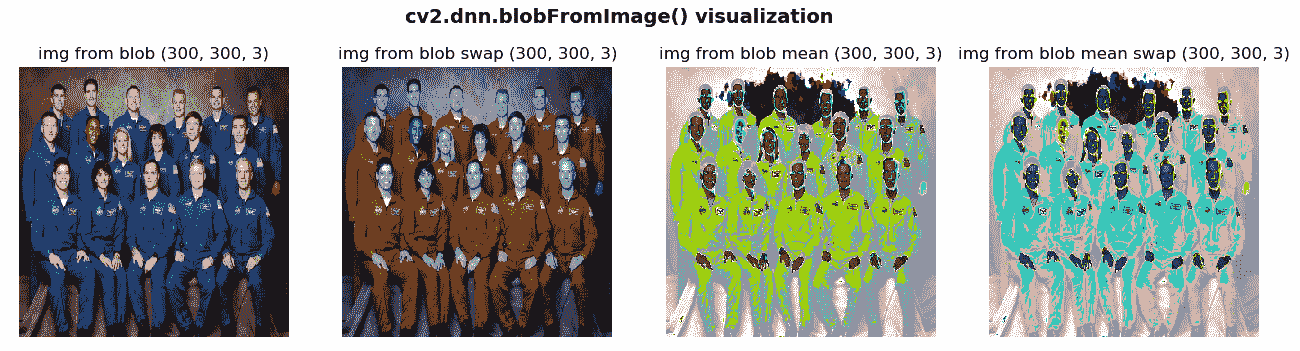blob_from_images.py脚本中，我们首先加载两个 BGR 图像，并使用cv2.dnn.blobFromImages()函数创建一个二维 BLOB。 您可以检查创建的 BLOB 的形状是否为(2, 3, 300, 300)。 然后，我们调用get_images_from_blob()函数，该函数可用于执行逆预处理转换，以便再次获取输入图像。

get_images_from_blob函数的代码如下：

def get_images_from_blob(blob_imgs, scalefactor, dim, mean, swap_rb, mean_added):
"""Returns images from blob"""

images_from_blob = cv2.dnn.imagesFromBlob(blob_imgs)
imgs = []

for image_blob in images_from_blob:
image_from_blob = np.reshape(image_blob, dim) / scalefactor
image_from_blob_mean = np.uint8(image_from_blob)
image_from_blob = image_from_blob_mean + np.uint8(mean)
if swap_rb:
image_from_blob = image_from_blob[:, :, ::-1]
imgs.append(image_from_blob)
else:
if swap_rb:
image_from_blob_mean = image_from_blob_mean[:, :, ::-1]
imgs.append(image_from_blob_mean)

return imgs


# Load images and get the list of images:
images = [image, image2]

# Call cv2.dnn.blobFromImages():
blob_images = cv2.dnn.blobFromImages(images, 1.0, (300, 300), [104., 117., 123.], False, False)
# The shape of the blob_image will be (2, 3, 300, 300):
print(blob_images.shape)

# Get different images from the blob:
imgs_from_blob = get_images_from_blob(blob_images, 1.0, (300, 300, 3), [104., 117., 123.], False, True)
imgs_from_blob_swap = get_images_from_blob(blob_images, 1.0, (300, 300, 3), [104., 117., 123.], True, True)
imgs_from_blob_mean = get_images_from_blob(blob_images, 1.0, (300, 300, 3), [104., 117., 123.], False, False)
imgs_from_blob_mean_swap = get_images_from_blob(blob_images, 1.0, (300, 300, 3), [104., 117., 123.], True, False)


• imgs_from_blob图像对应于调整为(300,300)尺寸的原始 BGR 图像。
• imgs_from_blob_swap图像对应于调整为(300,300)尺寸的原始 BGR 图像，并且蓝色和红色通道已交换。
• imgs_from_blob_mean图像对应于调整为(300,300)尺寸的原始 BGR 图像，其中带有平均值的标量尚未添加到图像。
• imgs_from_blob_mean_swap图像对应于调整为(300,300)的原始 BGR 图像，其中未将具有平均值的标量添加到图像中，并且蓝色和红色通道已交换。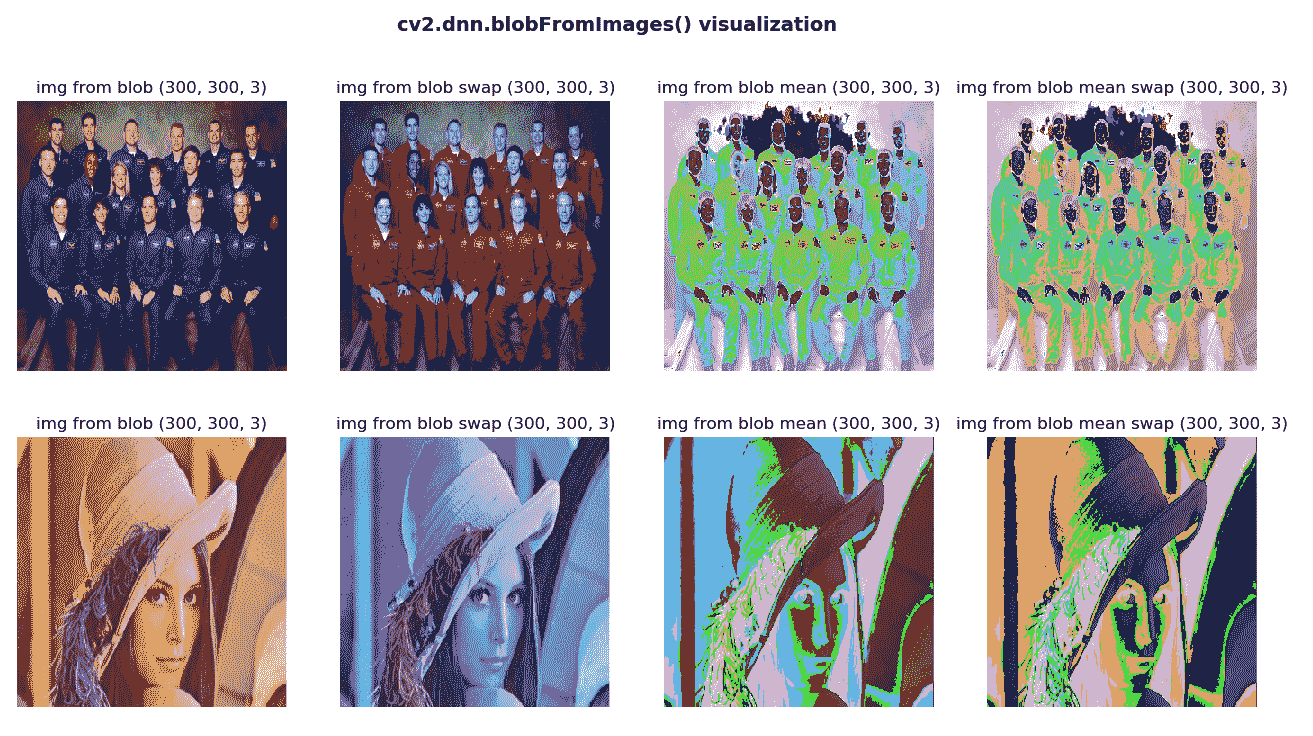cv2.dnn.blobFromImage()cv2.dnn.blobFromImages()的最后一个考虑因素是crop参数，该参数指示是否裁切图像。 在裁剪的情况下，图像将从中心裁剪，如以下屏幕截图所示：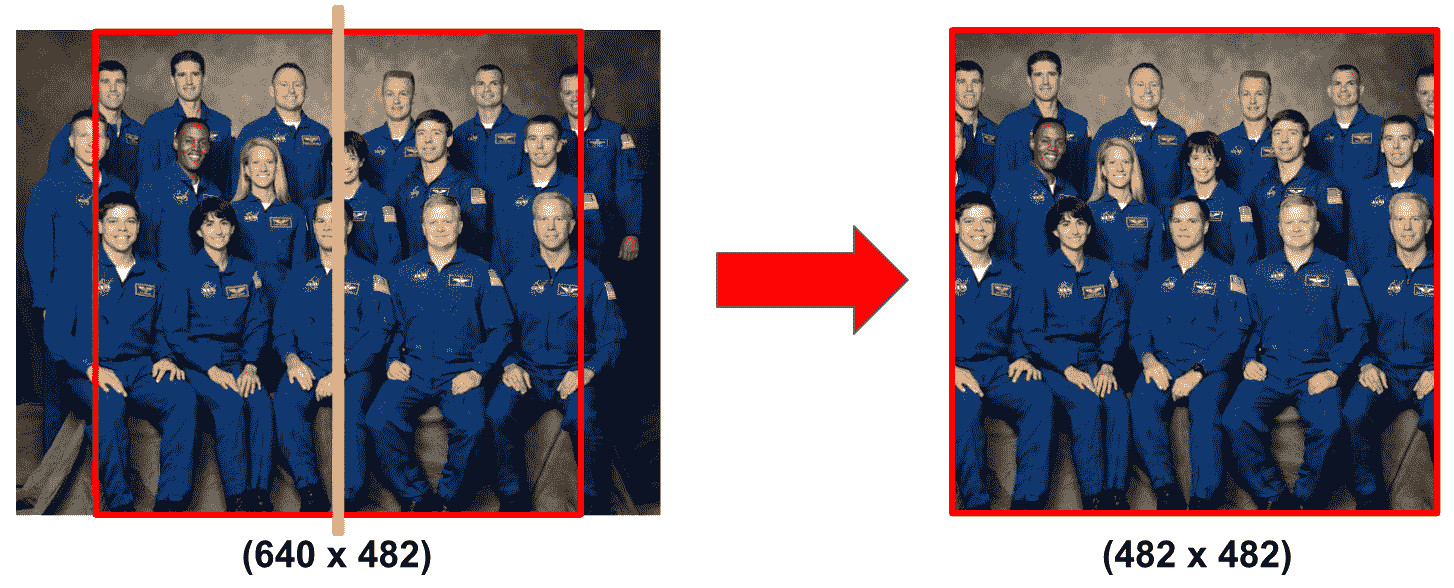def get_cropped_img(img):
"""Returns the cropped image"""

# calculate size of resulting image:
size = min(img.shape, img.shape)

# calculate x1, and y1
x1 = int(0.5 * (img.shape - size))
y1 = int(0.5 * (img.shape - size))

# crop and return the image
return img[y1:(y1 + size), x1:(x1 + size)]


blob_from_images_cropping.py脚本中，我们看到了裁剪的效果，并且还在get_cropped_img()函数中复制了裁剪过程：

# Load images and get the list of images:
images = [image, image2]

# To see how cropping works, we are going to perform the cropping formulation that
# both blobFromImage() and blobFromImages() perform applying it to one of the input images:
cropped_img = get_cropped_img(image)
# cv2.imwrite("cropped_img.jpg", cropped_img)

# Call cv2.dnn.blobFromImages():
blob_images = cv2.dnn.blobFromImages(images, 1.0, (300, 300), [104., 117., 123.], False, False)
blob_blob_images_cropped = cv2.dnn.blobFromImages(images, 1.0, (300, 300), [104., 117., 123.], False, True)

# Get different images from the blob:
imgs_from_blob = get_images_from_blob(blob_images, 1.0, (300, 300, 3), [104., 117., 123.], False, True)
imgs_from_blob_cropped = get_images_from_blob(blob_blob_images_cropped, 1.0, (300, 300, 3), [104., 117., 123.], False, True)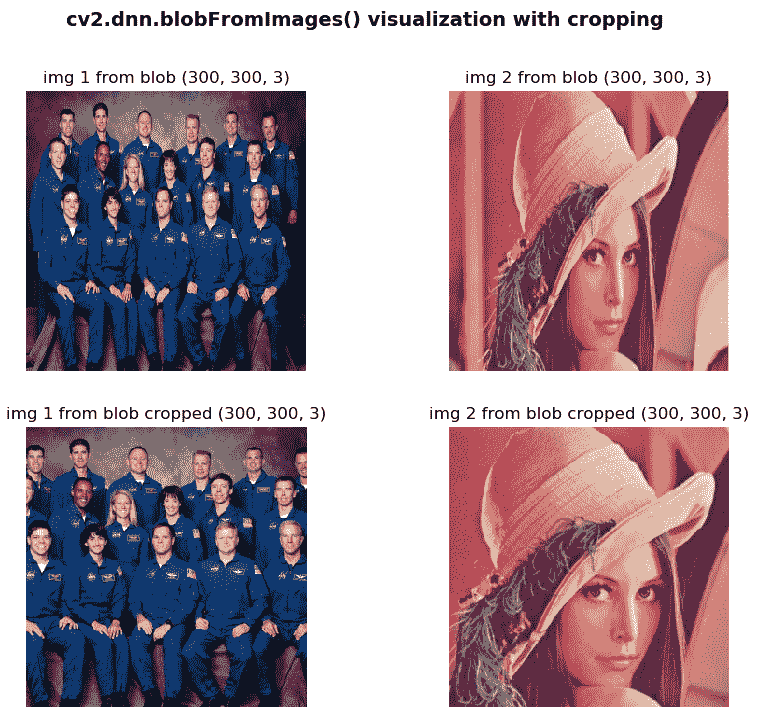# OpenCV DNN 人脸检测器的完整示例

• 当几张图像（可能具有不同的大小）馈送到网络时，计算输出– face_detection_opencv_cnn_images.py脚本
• cv2.dnn.blobFromImages()函数- face_detection_opencv_cnn_images_crop.py脚本中的crop=True参数馈入网络时，将几张图像（可能具有不同的尺寸）馈送到网络时，计算输出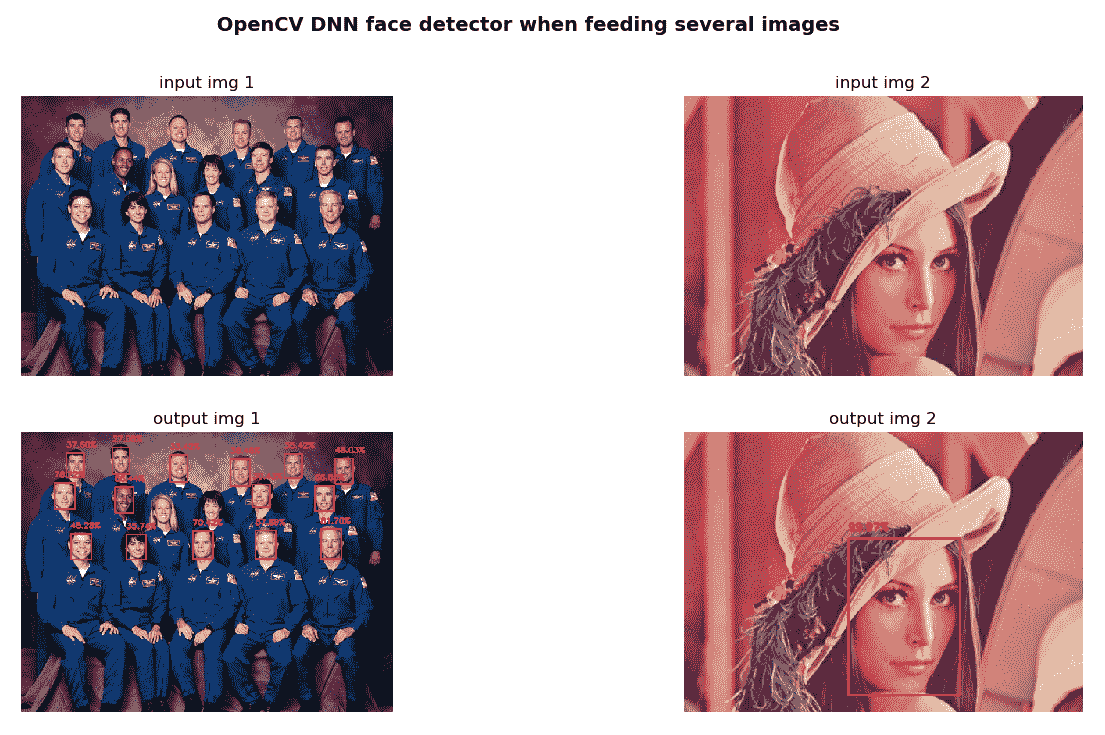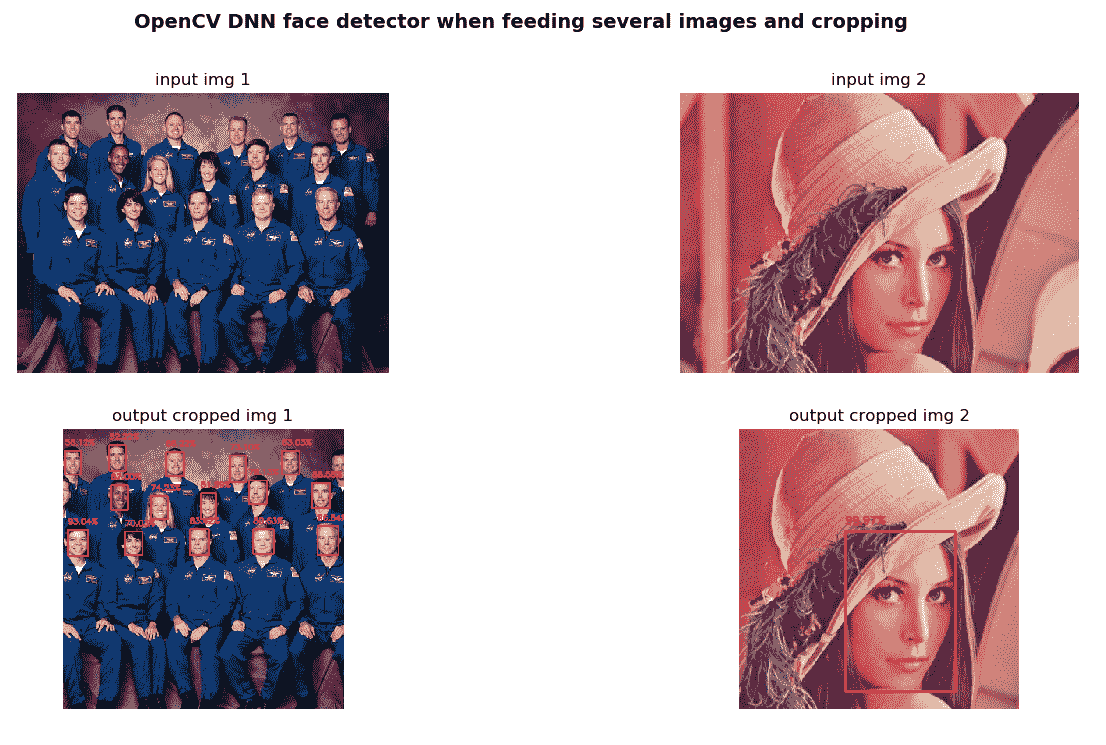# OpenCV 深度学习分类

# Feed the input blob to the network, perform inference and get the output:
net.setInput(blob)
preds = net.forward()

# Get inference time:
t, _ = net.getPerfProfile()
print('Inference time: %.2f ms' % (t * 1000.0 / cv2.getTickFrequency()))


net.getPerfProfile()方法返回推理的总时间和层的计时（以滴答为单位）。 这样，您可以使用不同的深度学习架构比较推理时间。

# 用于图像分类的 AlexNet

image_classification_opencv_alexnet_caffe.py脚本中，通过使用 AlexNet 和 Caffe 预训练模型，使用 OpenCV DNN 模块进行图像分类。 第一步是加载类的名称。 第二步是从磁盘加载序列化的 Caffe 模型。 第三步是加载输入图像进行分类。 第四步是创建大小为(227, 2327)(104, 117, 123)平均减法值的 BLOB。 第五步是将输入 BLOB 馈送到网络，执行推理并获得输出。 第六步是获得概率最高（降序排列）的 10 个索引。 这样，具有最高概率（最高预测）的索引将是第一个。 最后，我们将在图像上绘制与最高预测相关的类和概率。 在以下屏幕截图中可以看到此脚本的输出：• 1\. label: church, probability: 0.8325679898
• 2\. label: monastery, probability: 0.043678388
• 3\. label: mosque, probability: 0.03827961534
• 4\. label: bell cote, probability: 0.02479489893
• 5\. label: beacon, probability: 0.01249620412
• 6\. label: dome, probability: 0.01223050058
• 7\. label: missile, probability: 0.006323920097
• 8\. label: projectile, probability: 0.005275635514
• 9\. label: palace, probability: 0.004289720673
• 10\. label: castle, probability: 0.003241452388

text = "label: {} probability: {:.2f}%".format(classes[indexes], preds[indexes] * 100)
print(text)
y0, dy = 30, 30
for i, line in enumerate(text.split('\n')):
y = y0 + i * dy
cv2.putText(image, line, (5, y), cv2.FONT_HERSHEY_SIMPLEX, 0.8, (0, 255, 255), 2)


text = "label: {}\nprobability: {:.2f}%".format(classes[indexes], preds[indexes] * 100)• 1\. label: church, probability: 0.9082632661
• 2\. label: bell cote, probability: 0.06350905448
• 3\. label: monastery, probability: 0.02046923898
• 4\. label: dome, probability: 0.002624791814
• 5\. label: mosque, probability: 0.001077500987
• 6\. label: fountain, probability: 0.001011475339
• 7\. label: palace, probability: 0.0007750992081
• 8\. label: castle, probability: 0.0002349214483
• 9\. label: pedestal, probability: 0.0002306570677
• 10\. label: analog clock, probability: 0.0002107089822

# 用于图像分类的 ResNet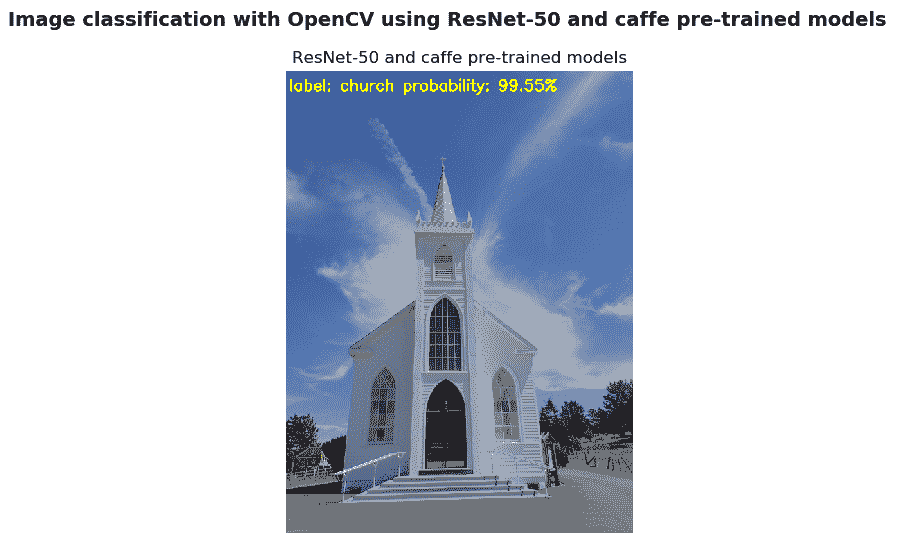• 1\. label: church, probability: 0.9955400825
• 2\. label: dome, probability: 0.002429900225
• 3\. label: bell cote, probability: 0.0007424423238
• 4\. label: monastery, probability: 0.0003768313909
• 5\. label: picket fence, probability: 0.0003282549733
• 6\. label: mosque, probability: 0.000258318265
• 7\. label: mobile home, probability: 0.0001083607058
• 8\. label: stupa, probability: 2.96174203e-05
• 9\. label: palace, probability: 2.621001659e-05
• 10\. label: beacon, probability: 2.02897063e-05

# 用于图像分类的 SqueezeNet

image_classification_opencv_squeezenet_caffe.py脚本中，我们使用 SqueezeNet 架构执行图像分类，该架构可将 AlexNet 级别的精度降低 50 倍。 在以下屏幕截图中可以看到此脚本的输出：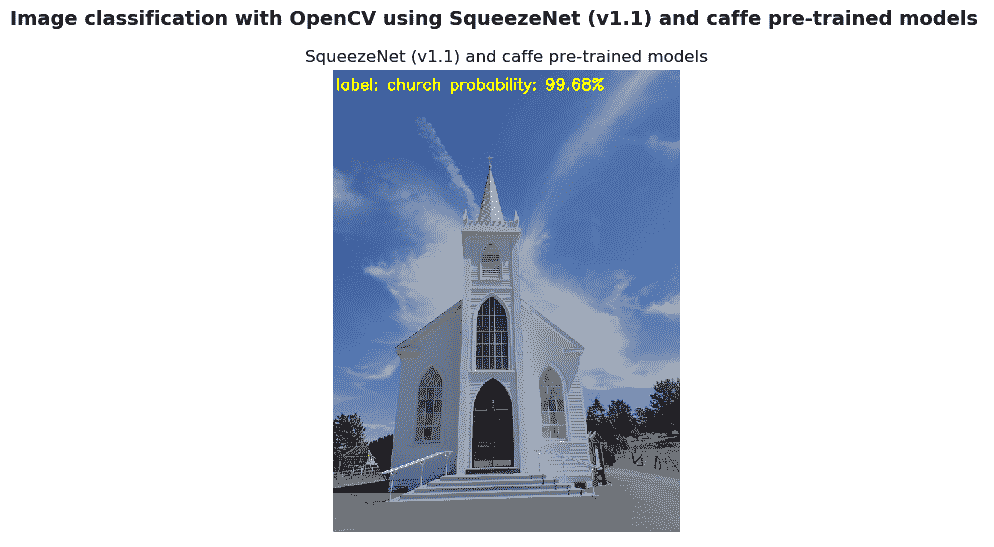• 1\. label: church, probability: 0.9967952371
• 2\. label: monastery, probability: 0.001899079769
• 3\. label: bell cote, probability: 0.0006924766349
• 4\. label: mosque, probability: 0.0002616141282
• 5\. label: dome, probability: 0.0001891527208
• 6\. label: palace, probability: 0.0001046952093
• 7\. label: stupa, probability: 8.239243471e-06
• 8\. label: vault, probability: 7.135886335e-06
• 9\. label: triumphal arch, probability: 6.732503152e-06
• 10\. label: cinema, probability: 4.201304819e-06

# 用于对象检测的 MobileNet-SSD

MobileNet-SSD 在 COCO 数据集上进行了训练，并在 PASCAL VOC 上进行了微调，达到了 72.27% 的 mAP（请参阅汇总 mAP 的表格以了解对象检测算法，以将该指标置于上下文中）。 在 PASCAL VOC 上进行微调时，可以检测到 20 个对象类，如下所示：

• ：人
• 动物：鸟，猫，牛，狗，马和羊
• 车辆：飞机，自行车，轮船，公共汽车，汽车，摩托车和火车
• 室内：瓶子，椅子，餐桌，盆栽，沙发和电视/显示器

object_detection_opencv_mobilenet_caffe.py脚本中，我们使用 OpenCV DNN 模块通过使用 MobileNet-SSD 和 Caffe 预训练模型来执行对象检测。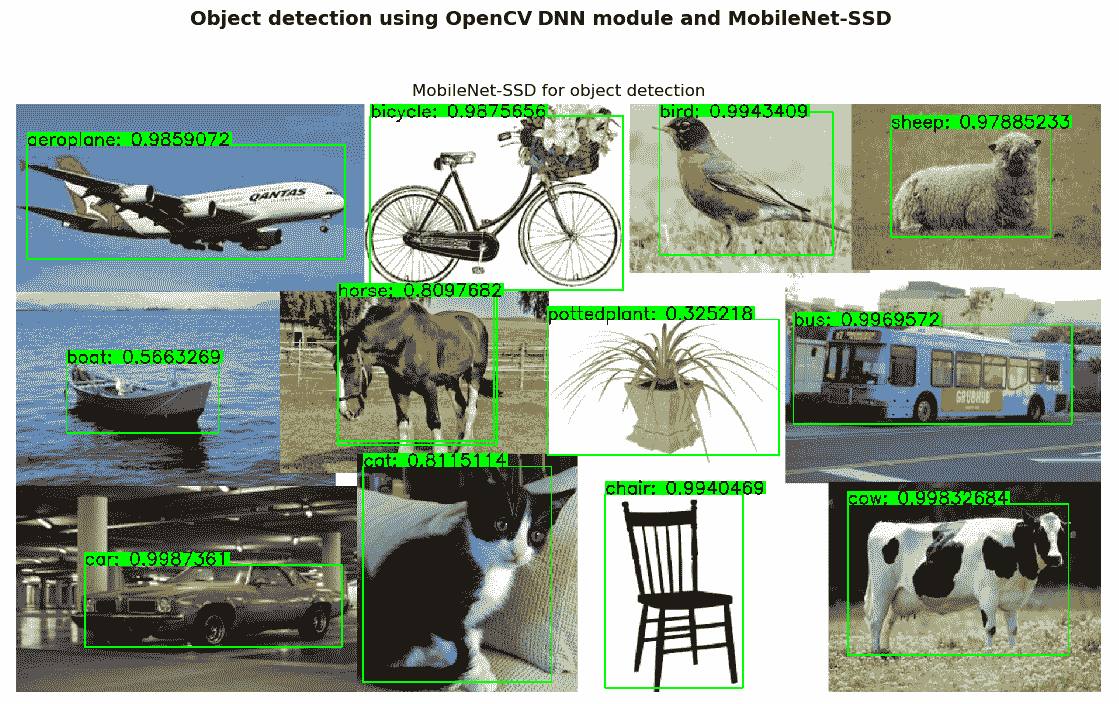# 用于对象检测的 YOLO

object_detection_opencv_yolo_darknet.py脚本中，使用 YOLO v3 进行对象检测。 YOLO v3 使用了一些技巧来改善训练并提高表现，其中包括多尺度预测和更好的主干分类器。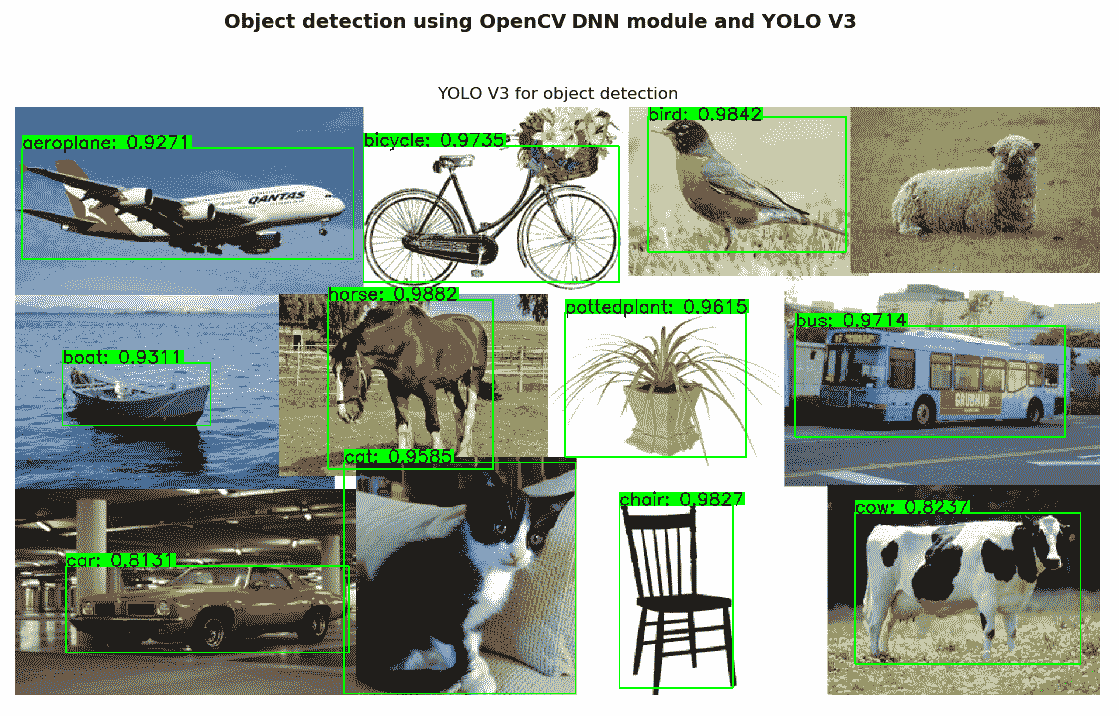# TensorFlow 库

TensorFlow 是 Google Brain 团队为内部使用而开发的用于机器学习和深度学习的开源软件平台。 随后，TensorFlow 于 2015 年在 Apache 许可下发布。在本节中，我们将看到一些示例，以向您介绍 TensorFlow 库。

# TensorFlow 的介绍示例

TensorFlow 库通过将操作链接到计算图中来表示要执行的计算。 创建此计算图后，您可以打开 TensorFlow 会话并执行该计算图以获取结果。 可以在tensorflow_basic_op.py脚本中看到此过程，该脚本执行在计算图中定义的乘法运算，如下所示：

# path to the folder that we want to save the logs for Tensorboard
logs_path = "./logs"

# Define placeholders:
X_1 = tf.placeholder(tf.int16, name="X_1")
X_2 = tf.placeholder(tf.int16, name="X_2")

# Define a multiplication operation:
multiply = tf.multiply(X_1, X_2, name="my_multiplication")


# Start the session and run the operation with different inputs:
with tf.Session() as sess:
summary_writer = tf.summary.FileWriter(logs_path, sess.graph)

print("2 x 3 = {}".format(sess.run(multiply, feed_dict={X_1: 2, X_2: 3})))
print("[2, 3] x [3, 4] = {}".format(sess.run(multiply, feed_dict={X_1: [2, 3], X_2: [3, 4]})))


\$ tensorboard --logdir="./logs"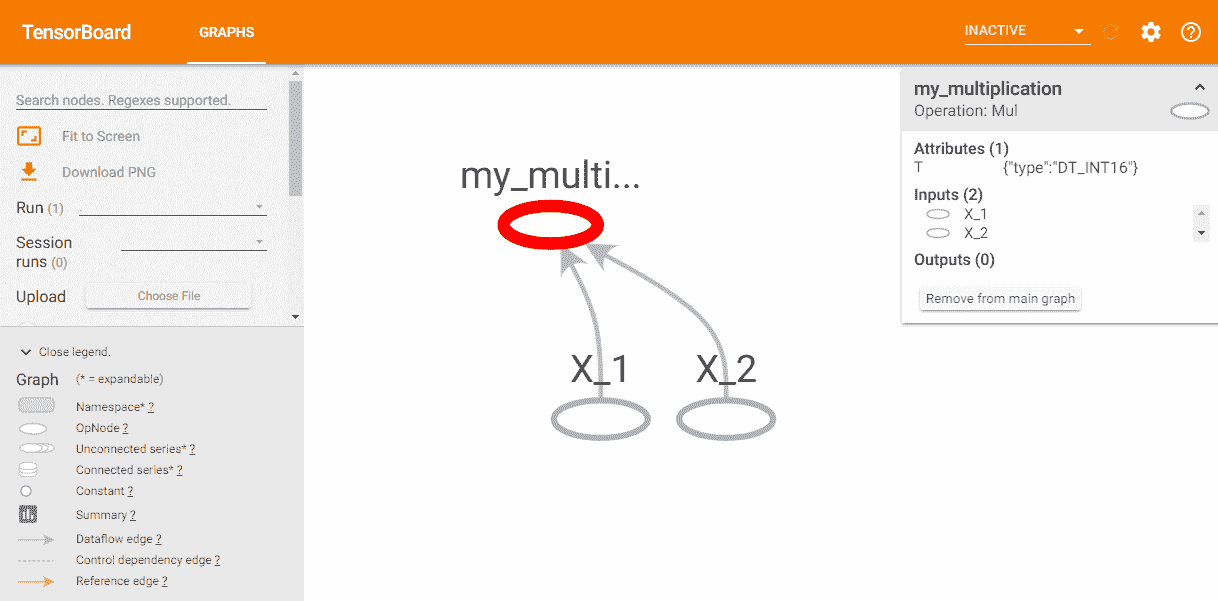with tf.name_scope('Operations'):
multiply = tf.multiply(X_1, X_2, name="my_multiplication")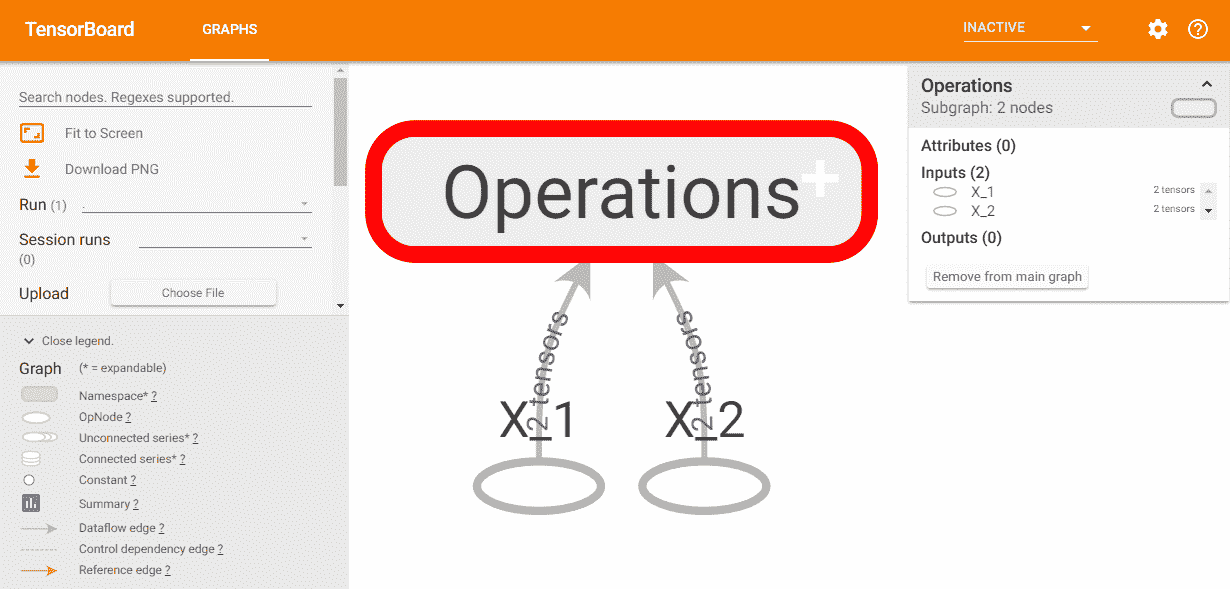# TensorFlow 中的线性回归

• tensorflow_linear_regression_training.py：此脚本生成线性回归模型。
• tensorflow_linear_regression_testing.py：此脚本加载创建的模型并使用它进行新的预测。
• tensorflow_save_and_load_using_model_builder.py：此脚本加载创建的模型，并使用SavedModelBuilder()导出模型以进行推断。 此外，此脚本还加载最终模型以做出新的预测。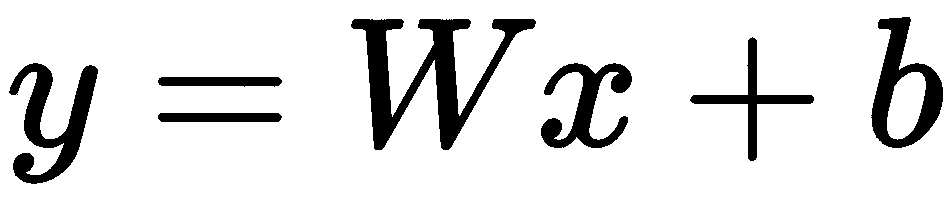x = np.linspace(0, N, N)
y = 3 * np.linspace(0, N, N) + np.random.uniform(-10, 10, N)


X = tf.placeholder("float", name='X')
Y = tf.placeholder("float", name='Y')


W = tf.Variable(np.random.randn(), name="W")
b = tf.Variable(np.random.randn(), name="b")


y_model = tf.add(tf.multiply(X, W), b, name="y_model")


cost = tf.reduce_sum(tf.pow(y_model - Y, 2)) / (2 * N)


optimizer = tf.train.GradientDescentOptimizer(learning_rate).minimize(cost)


init = tf.global_variables_initializer()


# Start the training procedure inside a TensorFlow Session:
with tf.Session() as sess:
# Run the initializer:
sess.run(init)

# Uncomment if you want to see the created graph
# summary_writer = tf.summary.FileWriter(logs_path, sess.graph)

# Iterate over all defined epochs:
for epoch in range(training_epochs):

# Feed each training data point into the optimizer:
for (_x, _y) in zip(x, y):
sess.run(optimizer, feed_dict={X: _x, Y: _y})

# Display the results every 'display_step' epochs:
if (epoch + 1) % disp_step == 0:
# Calculate the actual cost, W and b:
c = sess.run(cost, feed_dict={X: x, Y: y})
w_est = sess.run(W)
b_est = sess.run(b)
print("Epoch", (epoch + 1), ": cost =", c, "W =", w_est, "b =", b_est)

# Save the final model
saver.save(sess, './linear_regression')

# Storing necessary values to be used outside the session
training_cost = sess.run(cost, feed_dict={X: x, Y: y})
weight = sess.run(W)
bias = sess.run(b)

print("Training finished!")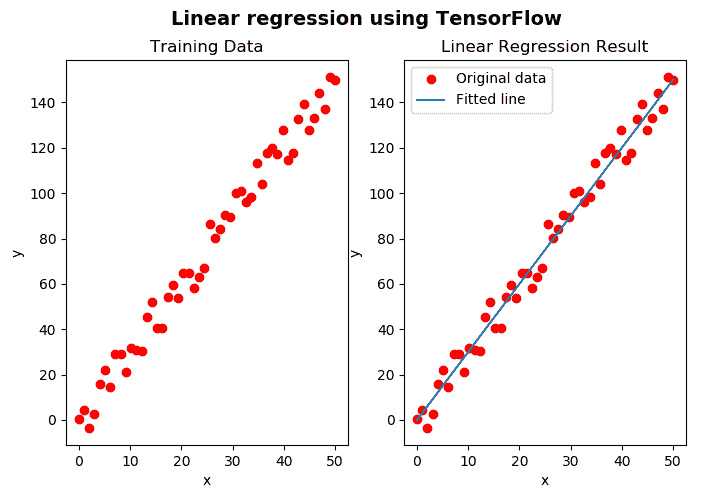• .meta文件：包含 TensorFlow 图
• .data文件：包含权重，偏差，梯度和所有其他已保存变量的值
• .index文件：标识检查点
• checkpoint文件：记录保存的最新检查点文件

tf.reset_default_graph()
imported_meta = tf.train.import_meta_graph("linear_regression.meta")


with tf.Session() as sess:
imported_meta.restore(sess, './linear_regression')
# Run the model to get the values of the variables W, b and new prediction values:
W_estimated = sess.run('W:0')
b_estimated = sess.run('b:0')
new_predictions = sess.run(['y_model:0'], {'X:0': new_x})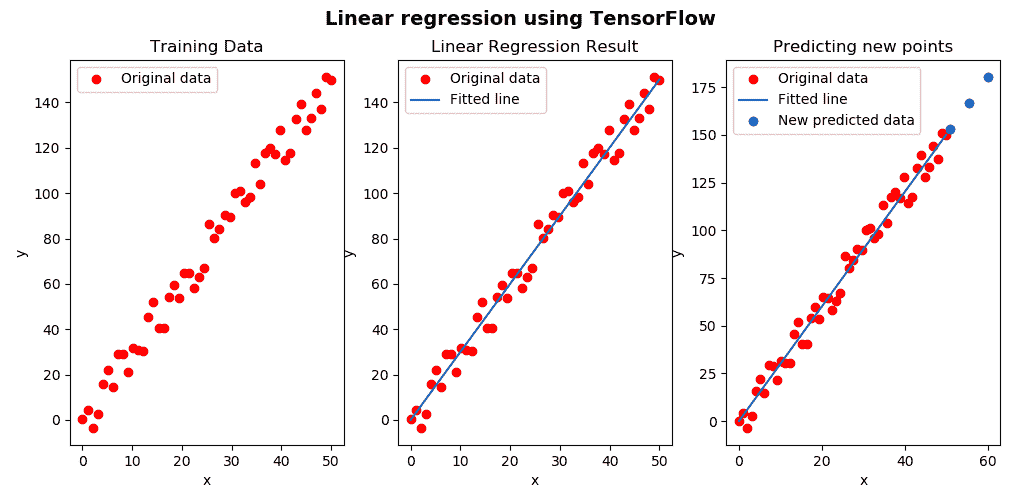def export_model():
"""Exports the model"""

trained_checkpoint_prefix = 'linear_regression'

sess.run(tf.global_variables_initializer())

# Restore from checkpoint

...
signature_map = {signature_constants.DEFAULT_SERVING_SIGNATURE_DEF_KEY: signature}

# Export model:
builder = tf.saved_model.builder.SavedModelBuilder('./my_model')
tags=[tf.saved_model.tag_constants.SERVING])
builder.save()


with tf.Session(graph=tf.Graph()) as sess:
graph = tf.get_default_graph()
x = graph.get_tensor_by_name('X:0')
model = graph.get_tensor_by_name('y_model:0')
print(sess.run(model, {x: new_x}))


# 使用 TensorFlow 的手写数字识别

Softmax 回归是可用于多类分类的逻辑回归的概括。 MNIST 数据集包含各种手写的数字图像：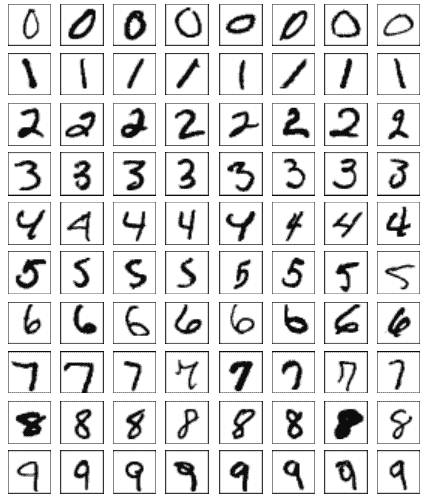mnist_tensorflow_save_model.py脚本创建用于学习和预测图像中手写数字的模型。

from tensorflow.examples.tutorials.mnist import input_data


x = tf.placeholder(tf.float32, shape=[None, 784], name='myInput')
y = tf.placeholder(tf.float32, shape=[None, 10], name='Y')


W = tf.Variable(tf.zeros([784, 10]))
b = tf.Variable(tf.zeros())


output_logits = tf.matmul(x, W) + b
y_pred = tf.nn.softmax(output_logits, name='myOutput')


tf.matmul()用于矩阵乘法，tf.nn.softmax()用于将softmax函数应用于输入张量，这意味着输出已归一化并且可以解释为概率。 在这一点上，我们可以定义损失函数，即创建优化器（在本例中为AdamOptimizer），模型的准确率如下：

# Define the loss function, optimizer, and accuracy
loss = tf.reduce_mean(tf.nn.softmax_cross_entropy_with_logits_v2(labels=y, logits=output_logits), name='loss')
correct_prediction = tf.equal(tf.argmax(output_logits, 1), tf.argmax(y, 1), name='correct_pred')
accuracy = tf.reduce_mean(tf.cast(correct_prediction, tf.float32), name='accuracy')


with tf.Session() as sess:
sess.run(tf.global_variables_initializer())
for i in range(num_steps):
# Get a batch of training examples and their corresponding labels.
x_batch, y_true_batch = data.train.next_batch(batch_size)

# Put the batch into a dict to be fed into the placeholders
feed_dict_train = {x: x_batch, y: y_true_batch}
sess.run(optimizer, feed_dict=feed_dict_train)

# Validation:
feed_dict_validation = {x: data.validation.images, y: data.validation.labels}
loss_test, acc_test = sess.run([loss, accuracy], feed_dict=feed_dict_validation)
print("Validation loss: {}, Validation accuracy: {}".format(loss_test, acc_test))

# Save model:
saved_path_model = saver.save(sess, './softmax_regression_model_mnist')
print('Model has been saved in {}'.format(saved_path_model))


# Load some test images:

with tf.Session(graph=tf.Graph()) as sess:
graph = tf.get_default_graph()
x = graph.get_tensor_by_name('myInput:0')
model = graph.get_tensor_by_name('myOutput:0')
output = sess.run(model, {x: [test_digit_0, test_digit_1, test_digit_2, test_digit_3]})
print("predicted labels: {}".format(np.argmax(output, axis=1)))


def load_digit(image_name):
"""Loads a digit and pre-process in order to have the proper format"""

gray = cv2.resize(gray, (28, 28))
flatten = gray.flatten() / 255.0
return flatten


predicted labels: [0 1 2 3]


# Keras 库

Keras 是用 Python 编写的开放源代码，高级神经网络 API（与 Python 2.7-3.6 兼容）。 它能够在 TensorFlow，Microsoft Cognitive Toolkit，Theano 或 PlaidML 之上运行，并且其开发重点是实现快速实验。 在本节中，我们将看到两个示例。 在第一个示例中，我们将看到如何使用与上一节中的 TensorFlow 示例相同的输入数据来解决线性回归问题。 在第二个示例中，我们将使用 MNIST 数据集对一些手写数字进行分类，就像在上一节中使用 TensorFlow 进行的操作一样。 这样，当解决相同类型的问题时，您可以清楚地看到两个库之间的差异。

# Keras 中的线性回归

linear_regression_keras_training.py数据集执行线性回归模型的训练。 第一步是创建用于训练/测试算法的数据，如下所示：

# Generate random data composed by 50 (N = 50) points:
x = np.linspace(0, N, N)
y = 3 * np.linspace(0, N, N) + np.random.uniform(-10, 10, N)


def create_model():
"""Create the model using Sequencial model"""

# Create a sequential model:
model = Sequential()
# All we need is a single connection so we use a Dense layer with linear activation:
# Compile the model defining mean squared error(mse) as the loss

# Return the created model
return model


linear_reg_model.fit(x, y, epochs=100, validation_split=0.2, verbose=1)


w_final, b_final = get_weights(linear_reg_model)


get_weights()函数返回这些参数的值，如下所示：

def get_weights(model):
"""Get weights of w and b"""

w = model.get_weights()
b = model.get_weights()
return w, b


# Calculate the predictions:
predictions = w_final * x + b_final


linear_reg_model.save_weights("my_model.h5")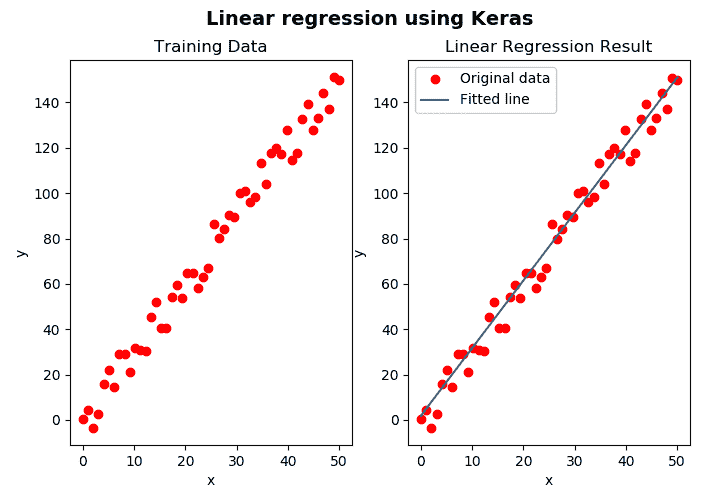linear_reg_model.load_weights('my_model.h5')


m_final, b_final = get_weights(linear_reg_model)


predictions = linear_reg_model.predict(x)
new_predictions = linear_reg_model.predict(new_x)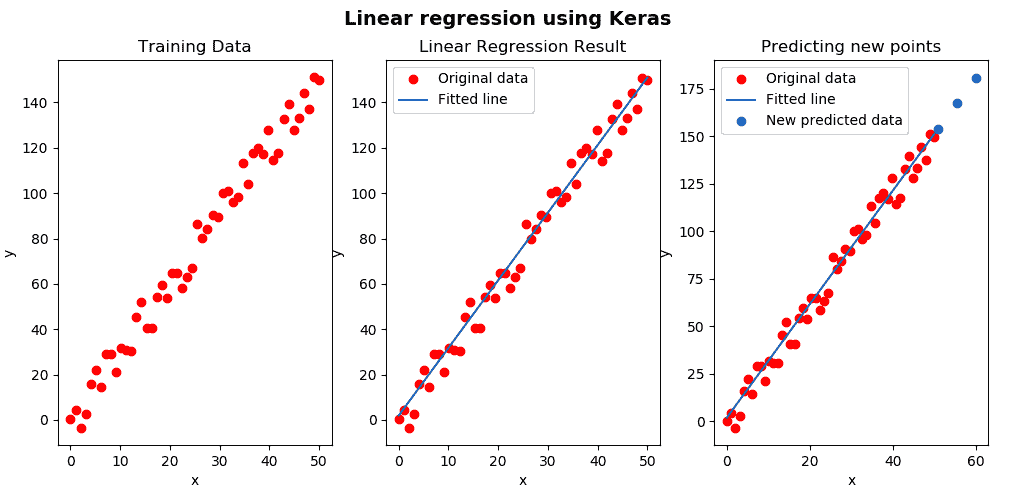# Keras 中的手写数字识别

def create_model():
"""Create the model using Sequencial model"""

# Create a sequential model (a simple NN is created) adding a softmax activation at the end with 10 units:
model = Sequential()

# Compile the model using the loss function "categorical_crossentropy" and Stocastic Gradient Descent optimizer:
model.compile(optimizer=SGD(0.001), loss="categorical_crossentropy", metrics=["accuracy"])

# Return the created model
return model


(train_x, train_y), (test_x, test_y) = mnist.load_data()


train_x = train_x.reshape(60000, 784)
test_x = test_x.reshape(10000, 784)
train_y = keras.utils.to_categorical(train_y, 10)
test_y = keras.utils.to_categorical(test_y, 10)


# Create the model:
model = create_model()

# Use the created model for training:
model.fit(train_x, train_y, batch_size=32, epochs=10, verbose=1)

# Save the created model:
model.save("mnist-model.h5")

# Get the accuracy when testing:
accuracy = model.evaluate(x=test_x, y=test_y, batch_size=32)

# Show the accuracy:
print("Accuracy: ", accuracy)


# Note: Images should have black background:
"""Loads a digit and pre-process in order to have the proper format"""

gray = cv2.resize(gray, (28, 28))
gray = gray.reshape((1, 784))

return gray

# Create the model:
model = create_model()

# Load parameters of the model from the saved mode file:

imgs = np.array([test_digit_0, test_digit_1, test_digit_2, test_digit_3])
imgs = imgs.reshape(4, 784)

# Predict the class of the loaded images
prediction_class = model.predict_classes(imgs)

# Print the predicted classes:
print("Class: ", prediction_class)



Class:  [0 1 2 3]


# 问题

1. 本章开头所述的机器学习和深度学习之间的三个主要区别是什么？
2. 哪一年被认为是深度学习的爆炸式增长？
3. 以下函数执行什么功能？ blob = cv2.dnn.blobFromImage(image, 1.0, (300, 300), [104., 117., 123.], False, False)
4. 以下几行执行什么操作？
net.setInput(blob)
preds = net.forward()

1. TensorFlow 中的占位符是什么？
2. 在 TensorFlow 中使用saver.save()保存模型时，将创建四个文件？
3. 单热编码是什么意思？
4. Keras 中的顺序模型是什么？
5. Keras 中model.fit()的作用是什么？